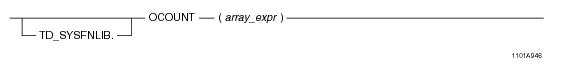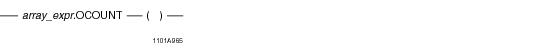# 15.00 - OCOUNT - Teradata Database

## Teradata Database SQL Functions, Operators, Expressions, and Predicates

Product
Release Number
15.00
Content Type
Programming Reference
Publication ID
B035-1145-015K
Language
English (United States)

## OCOUNT

### Purpose

Returns the number of elements in array_expr that contain either a NULL or non-NULL value.

### Syntax

System function syntax:Method-style syntax:where:

 Syntax element… Specifies… TD_SYSFNLIB the name of the database where the method is located. array_expr an ARRAY expression, which is one of the following: A reference to an ARRAY column An ARRAY constructor expression. See “ARRAY Constructor Expression” on page 195. A UDF expression A UDM expression

### ANSI Compliance

This is a Teradata extension to the ANSI SQL:2011 standard.

### Usage Notes

OCOUNT takes an array expression as an argument and returns the number of elements that contain a non-NULL value or NULL. Elements that are in an uninitialized state are not counted. If the array is empty (all elements of the array are in an uninitialized state), then OCOUNT returns a value of 0.

If array_expr is NULL, an error is returned.

The OCOUNT method is compatible with the Oracle COUNT method for one-dimensional ARRAY types. However, the empty set of parentheses required by Teradata syntax is a deviation from Oracle syntax.

### Result Type

OCOUNT returns an unsigned integer value.

### Example

Consider the following one-dimensional ARRAY data type and table.

`CREATE TYPE phonenumbers AS VARRAY(20) OF CHAR(10);`
` `
`CREATE TABLE employee_info (eno INTEGER, phonelist phonenumbers);`

The table is populated with the following values:

`/* The first 2 elements are populated; the rest are uninitialized. */`
`INSERT INTO employee_info VALUES (1, `
`   phonenumbers('1112223333', '6195551234'));`
` `
`/* Empty ARRAY instance */`
`INSERT INTO employee_info VALUES (2, `
`   phonenumbers());`
` `
`/* Update the empty ARRAY instance such that element 3 is set to a value; then elements 1 and 2 are set to NULL, the rest are uninitialized. */`
`UPDATE employee_info`
`SET phonelist = '8584850000'`
`WHERE id = 2;`

The following query returns the number of elements in the phonelist array that contain NULL or non-NULL values.

`SELECT eno, phonelist.OCOUNT() `
`FROM employee_info;`

The following is the result of the query.

`ENO         phonelist.OCOUNT()`
`---         ------------------`
` 1           2      (the first 2 elements have non-NULL values)`
` 2           3      (the first 3 elements have NULL or non-NULL values)`

### Example

Consider the following 2-D ARRAY data type and table.

`CREATE TYPE shot_ary AS VARRAY(1:50)(1:50) OF INTEGER;`
` `
`CREATE TABLE seismic_table (`
`   id INTEGER,`
`   shots shot_ary);`

The table is populated with the following values:

`/* The first 2 elements are populated; the rest are uninitialized. */`
`INSERT INTO seismic_table VALUES (1, shot_ary(11, 12));`
` `
`/* Empty ARRAY instance */`
`INSERT INTO seismic_table VALUES (2, shot_ary());`
` `
`/* Update the empty ARRAY instance such that element  is set to a value; then elements  and  are set to NULL, the rest are uninitialized */`
` `
`UPDATE seismic_table`
`SET shots = 1133`
`WHERE id = 2;`

The following query returns the number of elements in the shots array that contain NULL or non-NULL values.

`SELECT id, shots.OCOUNT()`
`FROM seismic_table;`

The following is the result of the query.

`ID          shots.OCOUNT()`
`--          --------------`
` 1           2    (the first 2 elements contain non-NULL values)`
` 2           3    (the first 3 elements contain NULL or non-NULL values)`

The following is the same query using function-style syntax.

`SELECT id, OCOUNT(shots) `
`FROM seismic_table;`